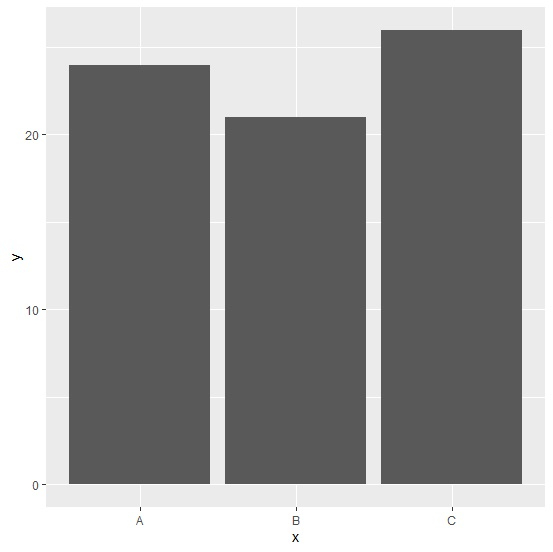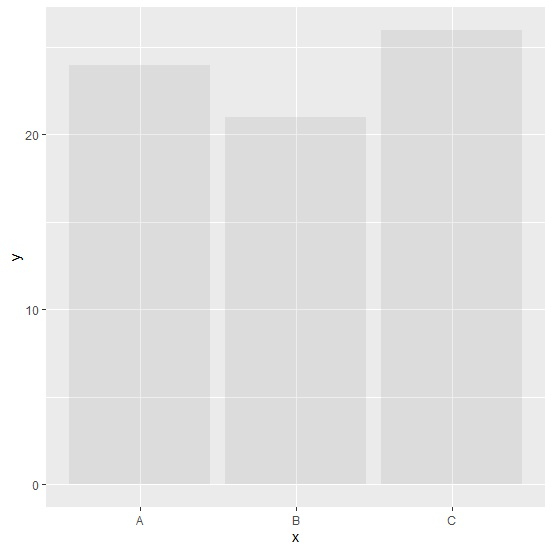# How to create transparent bar plot using ggplot2 in R?

To create transparent barplot using ggplot2, we can use alpha argument inside geom_bar function. For example, if we have a data frame called df that contains a categorical column say x and a numerical column say count then the bar plot with transparency can be created by using the command ggplot(df,aes(x,y))+geom_bar(alpha=0.1,stat="identity")

## Example

Consider the below data frame −

Live Demo

x<-c("A","B","C")
y<-c(24,21,26)
df<-data.frame(x,y)
df

## Output

   x  y
1  A  24
2  B  21
3  C  26

Loading ggplot2 package and creating bar plot for data in df −

## Example

library(ggplot2)
ggplot(df,aes(x,y))+geom_bar(stat="identity")

## OutputCreating bar plot for data in df with transparent bars −

## Example

ggplot(df,aes(x,y))+geom_bar(alpha=0.1,stat="identity")

## Output